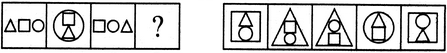# Non Verbal Reasoning - Analogy - Discussion

Discussion Forum : Analogy - Section 1 (Q.No. 13)
Directions to Solve

Each of the following questions consists of two sets of figures. Figures A, B, C and D constitute the Problem Set while figures 1, 2, 3, 4 and 5 constitute the Answer Set. There is a definite relationship between figures A and B. Establish a similar relationship between figures C and D by selecting a suitable figure from the Answer Set that would replace the question mark (?) in fig. (D).

13.

Select a suitable figure from the Answer Figures that would replace the question mark (?).(A)     (B)      (C)     (D)                  (1)      (2)      (3)      (4)      (5)
1
2
3
4
5
Explanation:
The rightmost element enlarges to become the outer element; the leftmost element becomes the inner-lower element and the middle element becomes the inner-upper element.
Discussion:
2 comments Page 1 of 1.

Ranjith said:   4 years ago
@Jane.

Add the figure right to left.

Jane said:   6 years ago
How did you get C as answer? Explain.